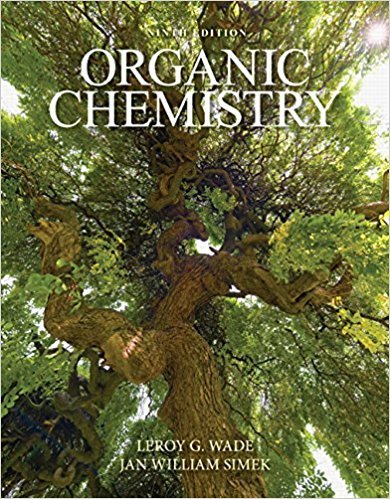×
×

# Solutions for Chapter 13: Nuclear Magnetic Resonance Spectroscopy## Full solutions for Organic Chemistry | 9th Edition

ISBN: 9780321971371Solutions for Chapter 13: Nuclear Magnetic Resonance Spectroscopy

Solutions for Chapter 13
4 5 0 313 Reviews
24
4
##### ISBN: 9780321971371

This textbook survival guide was created for the textbook: Organic Chemistry, edition: 9. Since 63 problems in chapter 13: Nuclear Magnetic Resonance Spectroscopy have been answered, more than 46673 students have viewed full step-by-step solutions from this chapter. This expansive textbook survival guide covers the following chapters and their solutions. Chapter 13: Nuclear Magnetic Resonance Spectroscopy includes 63 full step-by-step solutions. Organic Chemistry was written by and is associated to the ISBN: 9780321971371.

Key Chemistry Terms and definitions covered in this textbook
• alpha (a) rays.

Helium ions with a positive charge of 12. (2.2)

• Anti stereoselectivity

The addition of atoms or groups of atoms to opposite faces of a carbon-carbon double bond.

• anti-coplanar

A conformation in which a hydrogen atom and a leaving group are separated by a dihedral angle of exactly 180°.

• catenation.

The ability of the atoms of an element to form bonds with one another. (22.3)

• chemical changes

Processes in which one or more substances are converted into other substances; also called chemical reactions. (Section 1.3)

• coordination number.

In a crystal lattice it is defined as the number of atoms (or ions) surrounding an atom (or ion) (11.4). In coordination compounds it is defined as the number of donor atoms surrounding the central metal atom in a complex. (23.3)

• cubic close packing

A crystal structure where the atoms are packed together as close as possible, and the close-packed layers of atoms adopt a three-layer repeating pattern that leads to a face-centered cubic unit cell. (Section 12.3)

• diene

A compound containing two carbon-carbon p bonds.

• Enol

A compound containing a hydroxyl group bonded to a doubly bonded carbon atom.

• equilibrium-constant expression

The expression that describes the relationship among the concentrations (or partial pressures) of the substances present in a system at equilibrium. The numerator is obtained by multiplying the concentrations of the substances on the product side of the equation, each raised to a power equal to its coefficient in the chemical equation. The denominator similarly contains the concentrations of the substances on the reactant side of the equation. (Section 15.2)

• fishhook arrow

A curved arrow with only one barb, indicating the motion of just one electron (also see Sect. 11.1).

• Haber process

The catalyst system and conditions of temperature and pressure developed by Fritz Haber and coworkers for the formation of NH3 from H2 and N2. (Section 15.2)

• Hemiacetal

A molecule containing an !OH and an !OR or !OAr group bonded to the same carbon

• Lewis base

Any molecule or ion that can form a new covalent bond by donating a pair of electrons.

• ligand

An ion or molecule that coordinates to a metal atom or to a metal ion to form a complex. (Section 23.2)

• nucleophilic attack

One of the four arrow-pushing patterns for ionic reactions.

• octet rule

A rule stating that bonded atoms tend to possess or share a total of eight valence-shell electrons. (Section 8.1)

• Plane of symmetry

An imaginary plane passing through an object dividing it so that one half is the mirror image of the other half

• Racemic mixture

A mixture of equal amounts of two enantiomers.

• Z (Section 5.2C)

From the German, zusammen, meaning opposite. Specifi es that groups of higher priority on the carbons of a double bond are on the same side

×